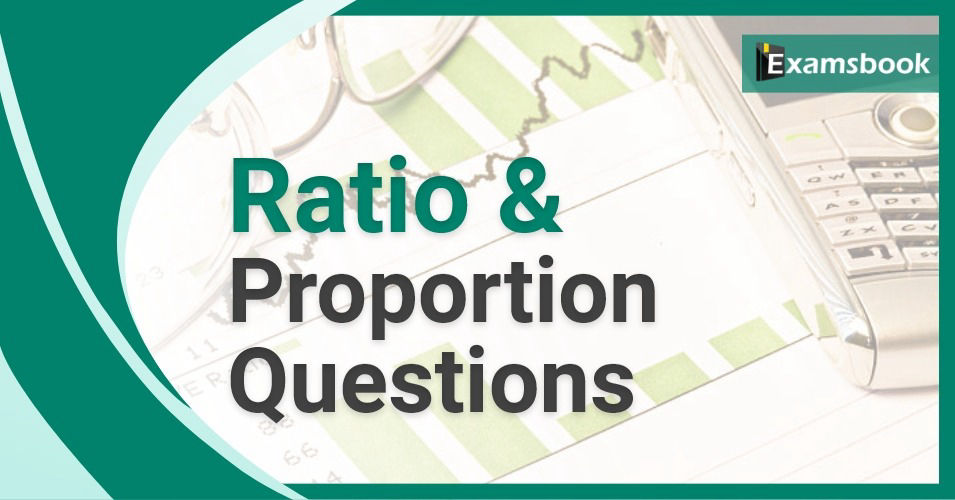• Save

# Ratio and Proportion Questions and Answers Aptitude Problems

Rajesh Bhatia10 months ago 50.3K ViewsRatio and Proportion Questions are important for competitive exams point of view. Here I am providing Ratio and Proportion questions and answers for your practice. So let's practice with selective Ratio and Proportion Questions and answers for competitive exams like SSC and Banking exams.

If you don't want to face any difficulty related ratio and proportion problems, you should practice ratio and proportion problems with solutions for ssc and bank exams.

Students can solve easily these questions if they know ratio and proportion formulas that how to use formulas in these questions.

## Ratio and Proportion Questions and Answers for Competitive Exams

Q.1 If (4x-3y) : (2x+5y) = 12 : 19 then x : y is…….

(A) 2:3

(B) 1 : 2

(C) 3 : 2

(D) 2 : 1

Ans .  C

To know more about Time and Work: Time and work aptitude questions for competitive exams

Q.2 If x/5 = x/8, then (x +5) : (x + 8) is equal to……

(A) 3 : 5

(B) 13:8

(C) 8:5

(D) 5:8

Ans .  D

Q.3 If x : y = 6 : 5, then (5x+3y) : (5x-3y) is equal to……

(A) 2 : 1

(B) 3 : 1

(C) 5 : 3

(D) 5 : 2

Ans .  B

Q.4 What same number must be added to each term of the ratio 7 : 3 so that the ratio becomes 2 : 3?

(A) 1

(B) 2

(C) 5

(D) can’t be determined

Ans .  D

Q.5 The ratio of the two numbers is 3 : 4 and their sum is 420. The greater of the two numbers is……

(A) 175

(B) 200

(C) 240

(D) 315

Ans .  C

Q.6 Five bananas and four apples cost as much s three bananas and seven apples. The ratio of the cost of one banana to that of one apple is…….

(A) 3 : 2

(B) 4 : 3

(C) 3 : 4

(D) 1 : 3

Ans .  A

Q.7 The speeds of three cars are in the ratio 5 : 4 : 6 . The ratio between the times taken by them to travel the same distance is…….

(A) 5 : 4 : 6

(B) 6 : 4 : 5

(C) 10 : 12 : 15

(D) 12 : 15 : 10

Ans .  D

Q.8 A dog takes 3 leaps for every 5 leaps of a hare. If one leap of the dog is equal to 3 leaps of the hare, the ratio of the speed of the dog to that of the hare……

(A) 8 : 5

(B) 9 : 5

(C) 8 : 7

(D) 9 : 7

Ans .  B

Q.9 Rs. 180 contained in a box consists of one rupee, 50 paise and 25 paise coins in the proportion of 2 : 3 : 4. What is the number of 50 paise coins?

(A) 120

(B) 150

(C) 180

(D) 240

Ans .  A

Q. 10 In a school, 10% of the boys are same in number as ¼ of the girls and 10% of the girls are same in number as 1/25 of the boys. What is the ratio of boys to girls in that school?

(A) 3 : 2

(B) 5 : 2

(C) 2 : 1

(D) 4 : 3

Ans .  B

### Rajesh Bhatia

A Writer, Teacher and GK Expert. I am an M.A. & M.Ed. in English Literature and Political Science. I am highly keen and passionate about reading Indian History. Also, I like to mentor students about how to prepare for a competitive examination. Share your concerns with me by comment box. Also, you can ask anything at linkedin.com/in/rajesh-bhatia-7395a015b/.

## Report Error: Ratio and Proportion Questions and Answers Aptitude Problems